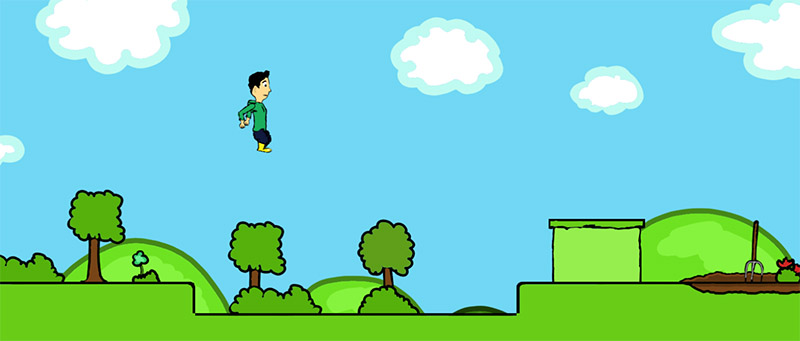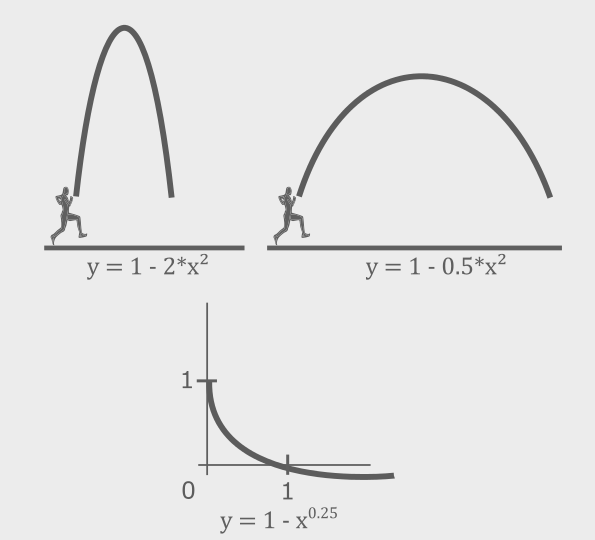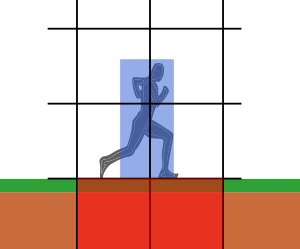# A technical description of 2D platformers

The 2D platformer enjoyed its major hype back in the 90s with blockbuster series like Super Mario, Mega Man, Sonic, Donkey Kong Country and many, many more. Nowadays the genre is witnessing kind of a renaissance, mostly through the new range of indie developers.

In this article I try to cover the major technical aspects on implementing a 2D platformer game.

## The Level

The level is the main component of the game. It basically consists of two parts:

• The terrain,
• A list of actors, so-called sprites.

### Terrain

The terrain can be built upon different layers of tiles. A common way is to use square tiles aligned on a grid. The terrain is the static component of the level and is usually not changing (with the exception of destructible terrain).

The tileset is a texture which contains all tiles usually for a specific theme. The tile size describes the size of the individual tiles and is a frequently used value in game logic and drawing calculations.

Each tile has in its most basic form three attributes: an index which tells what tile of the tileset should be drawn, and a value which describes the interaction when colliding with other objects. When there are multiple tilesets used, there has to be a third attribute which tells what tileset to use.

### Sprites

The term “sprite” is used in computer graphics to simply describe a texture that is drawn on a specific position. I will go a little bit further and use the term to describe any dynamic object in the level which are mainly enemies, hazards and other mechanics or, generally said, objects that move or perform any action.

Besides the texture and position attributes a sprite has a function which executes the individual behavior. Because there are a lot of routines which are commonly required by most sprites, it’s recommended that there is some kind of a base class for sprites which can then be overridden in order to implement more specific functionality.

Here’s an example:

```class BaseSprite {
// basic sprite properties
vector2d position
int width
int height
texture2d texture

// behavior parameters
float     movingSpeed
bool      isMoving
vector2d  movingDirection

update() {
// Common sprite functionalty
if (isMoving) {
velocity = movingDirectory * movingSpeed
}
// ...
}
}

class DashingEnemy : BaseSprite {
// specific properties
float dashSpeed

DashingEnemy(vector2 position) {
isMoving = true
movingSpeed = 10
}

override update() {

// specific sprite functionality
movingDirection = normalize(player.position - position)
if (abs(player.position - position) < 200) {
movingSpeed = dashSpeed
}

// call base method
base.update()
}
}
```

## The Player

The player is a special character which the actual player is controlling. The player’s abilities can vary greatly but at the very least he will be able to move around. In order to live up to the genre’s name the player can most likely jump and run – two moves which should be worked out carefully.

Good controls of the player’s character are among the most important factors of any game. That’s why you should put enough effort into the player’s physics which can mean a lot of playing around and tweaking until it feels perfect.

The player’s physics should be developed at an early stage in development and finished as soon as possible. Making changes in the player’s physics when there are already levels created could eventually mess up the whole level design.

The player has similar properties with the sprite, in fact the player could be considered as a type of sprite. Therefore the Player class can be derived from the Sprite class but it’s not necessary. Generally the player’s Update-Method can be divided into the following actions:

• Getting the player’s input from the keyboard/gamepad
• Applying the physics
• Collision detection

Let’s take a look at those in detail.

### Getting the input

The ways of getting device input depend on the platform and framework you are using. Most modern frameworks/engines provide a relatively easy way of handling input however.

Other than that the input handling should usually be pretty simple. The running direction can directly be set depending on the particular direction buttons. Any additional actions like jumping, ducking or shooting can be set via a flag which will be used later in the code.

```GetInput() {
if (isKeyDown(Left))
direction.x = -1
if (isKeyDown(Right))
direction.x = 1

isJumping = isKeyDown(Up)
isDucking = isKeyDown(Down)
}
```

### Applying the physics

There is no definitive way how to implement the player’s physics as it depends on the type of gameplay you want. The physics can range from scientifically accurate to completely unrealistic and arcade. Still, in both cases it is useful to rely on the basic laws of science and use the formulas we all learned in school. The most important of all is Newton’s second law of motion:  F = m * a, and derived from that the equation of acceleration and velocity: v = a * t.

First we determine the horizontal velocity and the basic vertical velocity which will be the gravity.

```velocity.x = direction.x * acceleration * deltaTime
velocity.y = gravityAcceleration * deltaTime
```

Next we will add the jumping routine. In case of a jump the vertical velocity gets overridden by the jumping curve. Here we have to take a little detour to geometry and utilize our knowledge of parabolic curves as they describe a jump best. We take the basic formula y = x² and invert it first by subtracting the whole term from 1 (y = 1 – x²). Then we add a multiplication coefficient to x depending on how our curve should look like. A smaller coefficient means a flatter curve while a higher means a steep curve.

The bad news is that we can’t use this exact formula directly in our code because we don’t need the Y-position but rather the player’s vertical velocity at any time. The curve of the veloctiy is again inverted, having its highest values at the start and the end of the jump and its minimum at the apex of the jump when the player is “floating” for a little moment. The formula for this curve could look something like 1 – x0.25, whereas x represents the current time of the jump, being a value from 0 to 1 (current time of the jump divided by the maximum jump time).

```velocity.y = launchVelocity * (1 - pow(jumpTime / maxJumpTime, 0.25));
```

There are two parameters in this term you may have to tweak until the jump feels like expected.

• The launch velocity is the initial power with which the player is shot into the air. Due to the nature of the jump this will be a high negative value (about -2000, depending on the other properties).
• The maximal jump time determines the time until the player reaches the apex of the jump, when making full use of the whole jump. When trying to achieve kind of realistic physics, this will be a relatively short period of time (< 1 sec). A longer maximal jump time means a higher control of the jumping height, depending on how long the player keeps the jump button pressed.Here’s a code overview of the entire jumping routine:

```Jump() {
if (isJumping) // if jump button is pressed
{
// Start or continue the jump
if ((!wasJumping && isOnGround) || jumpTime > 0)
jumpTime += deltaTime

// During the jump
if (0 < jumpTime && jumpTime <= maxJumpTime)
{
velocity.y = launchVelocity * (1 - pow(jumpTime / maxJumpTime, 0.25))
}
else
{
// reached apex of the jump
jumpTime = 0
}
}
else
{
// cancel jump / don't jump
jumpTime = 0
}
wasJumping = isJumping
}
```

After all velocity-affecting things have been calculated we can finally update the player’s actual position. That’s done by simply adding the velocity vector to the position and after that rounding it to get integer screen coordinates out of the floating point vectors.

```position += velocity
position = new vector2(round(position.x), round(position.y))
```

Now that we have elaborately calculated the player’s position, we may or may not have to revert it doing the collision detection.

### Collision detection

In the act of collision detection we check if the player is colliding with any other sprite or any tile in the level at the moment. This is done by using rectangular bounding boxes and checking if any of those are intersecting with each other. In this section we can also utilize one of the major advantages of our tile-based level setup.

We will separate the process in two parts. Checking if the player collides with a tile and checking if the player collides with a sprite.

Let’s start with the tiles. Thanks to our tile grid we don’t have to check the entire level’s tiles but can easily determine the player’s neighbors, which are the only relevant.

```int leftTile = floor(bounds.left / Tilesize)
int rightTile = ceiling(bounds.right / Tilesize) - 1
int topTile = floor(bounds.top / Tilesize)
int bottomTile = ceiling(bounds.bottom / Tilesize) - 17

for (int y = topTile; y <= bottomTile; y++)
for (int x = leftTile; x <= rightTile; x++)
// Do collision checking
```We loop through the neighbor tiles and perform the collision detection routine for each one. At the beginning of each iteration we retrieve the touch interaction value of the current tile. The most basic constants for this value would be solid and passable. If the tile is passable, there is no way of interaction so we can skip the collision detection. Otherwise we take further action. In the next step we process the actual intersection between the player’s bounding box and the tile’s box. What we need is the depth of the intersection, so the size of the overlapping part of the two rectangles, as well as the side of the player’s bounding box that intersects with the tile.

Now with these two values we should be able to perform any desired collision checks, most importantly checking if the player is standing on the ground.

After that we have to resolve the collision to prevent the player from going through the wall or the floor. This is done by simply adding the intersection depth to the player’s position.

```int collision = GetCollision(x, y)
if (collision != Blocks.Passable) {
vector2 depth = GetIntersectionDepth(tileRect)
int side = GetCollisionSide(tileRect)

// Do block specific collision action
collision = DoBlockCollision(x, y, collision, side, depth)

if (depth != vector2.zero) {
//// Kollision entlang der Y-Achse ////

// Player reached the top of the tile, so he is standing
if (side == Collision.BOTTOM && collision == Blocks.Solid)
isOnGround = true;

// Player bumped his head at the bottom of the tile, so the jump is aborted
if (side == Collision.TOP && collision == Blocks.Solid) {
// Resolve collision on the y-axis
position = new vector2(position.x, position.y + depth.y)

// Update bounding box for further calculations
bounds = GetBounds()

// Abort the jump
jumpTime = 0
}

// Player is on ground
if (isOnGround) {
// Resolve collision on the y-axis
position = new vector2(position.x, position.y + depth.y)

// Update bounding box for further calculations
bounds = GetBounds()
}

// Player hit the left or right side of the tile
if (collision == Blocks.Solid && (side == Collision.LEFT || side == Collision.RIGHT)) {
// Resolve collision on the x-axis
position = new vector2(position.x + depth.x, position.y)

// Update bounding box for further calculations
bounds = GetBounds()
}
}
}
```

Now that this is done, let’s head to the sprites. This part is far more straightforward because there’s no other way than checking collision with any sprite in the level. Because we don’t want to do the rather costly collision calculation unnecessarily though, we first check if the sprite is actually in range of the player.

```foreach (sprite in level.sprites) {
if (sprite.isInRange(player)) {
int playerCollision = GetCollision(sprite.bounds)

if (playerCollision != Collision.NONE) {
// Do sprite specific collision action
sprite.OnPlayerCollision(playerCollision, player)
}
}
}
```